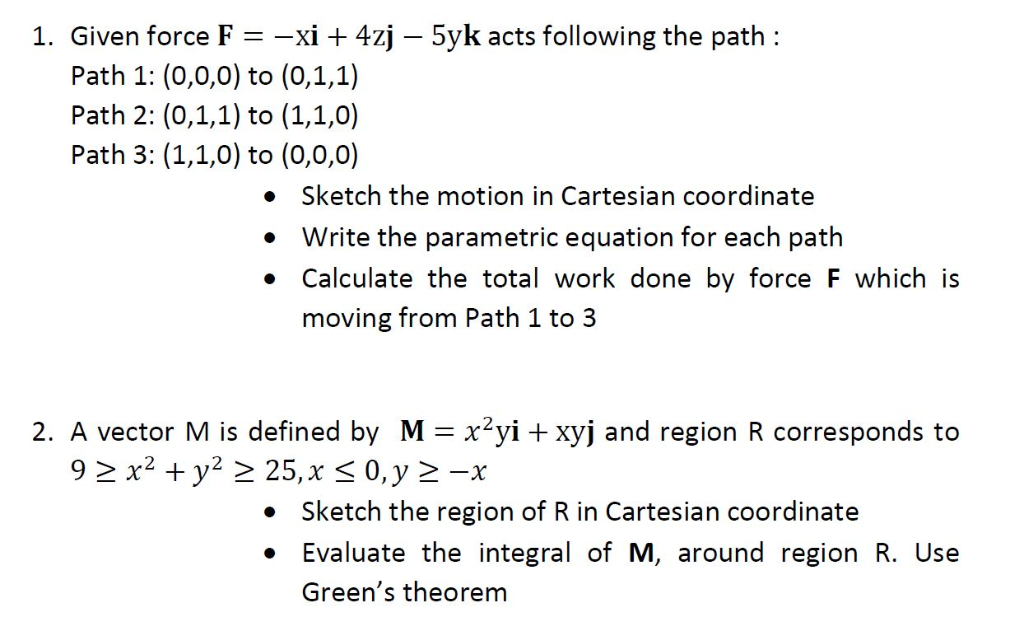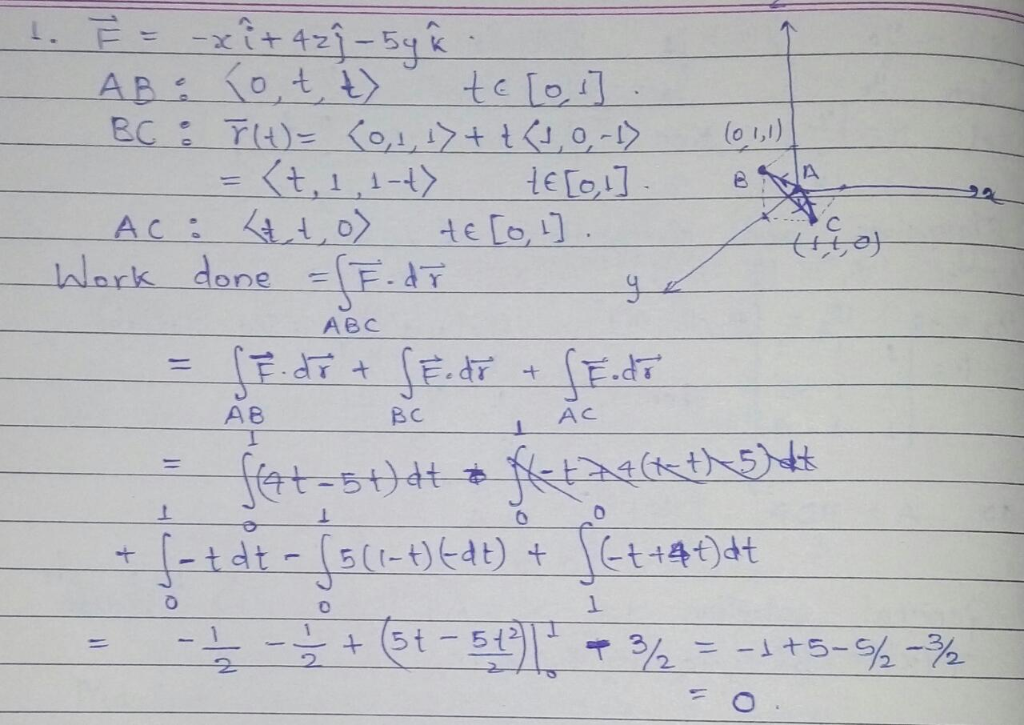# 1. Given force F-xi 4zj Path 1: (0,0,0) to (0,1,1) Path 2: (0,1,1) to (1,1,0) Path 3: (1,1,0) to (0,0,0) 5yk acts follo...1. Given force F-xi 4zj Path 1: (0,0,0) to (0,1,1) Path 2: (0,1,1) to (1,1,0) Path 3: (1,1,0) to (0,0,0) 5yk acts following the path: . Sketch the motion in Cartesian coordinate Write the parametric equation for each path Calculate the total work done by force F which is moving from Path 1 to 3 2. A vector M is defined by M-x2yi xyj and region R corresponds to Sketch the region of R in Cartesian coordinate . Evaluate the integral of M, around region R. Use Green's theoremfirst complete question according to chegg policy.

##### Add Answer of: 1. Given force F-xi 4zj Path 1: (0,0,0) to (0,1,1) Path 2: (0,1,1) to (1,1,0) Path 3: (1,1,0) to (0,0,0) 5yk acts follo...
More Homework Help Questions Additional questions in this topic.